Examples

Chapter 6 Class 12 Application of Derivatives
Serial order wise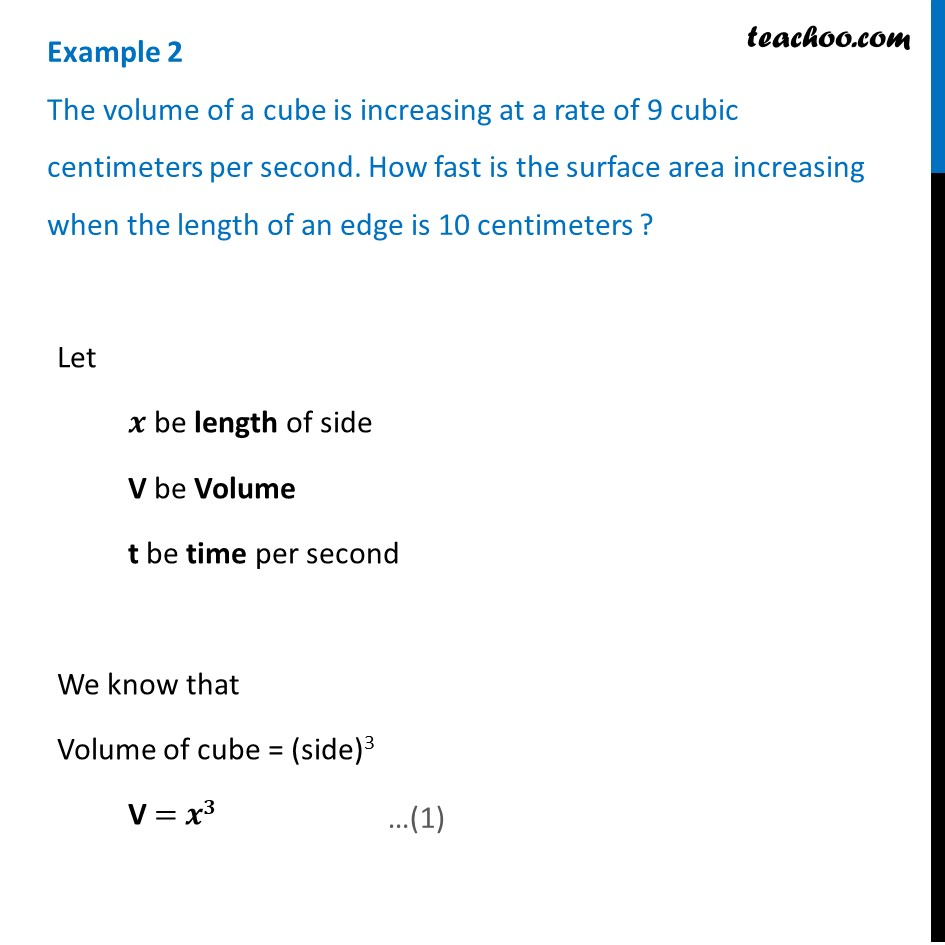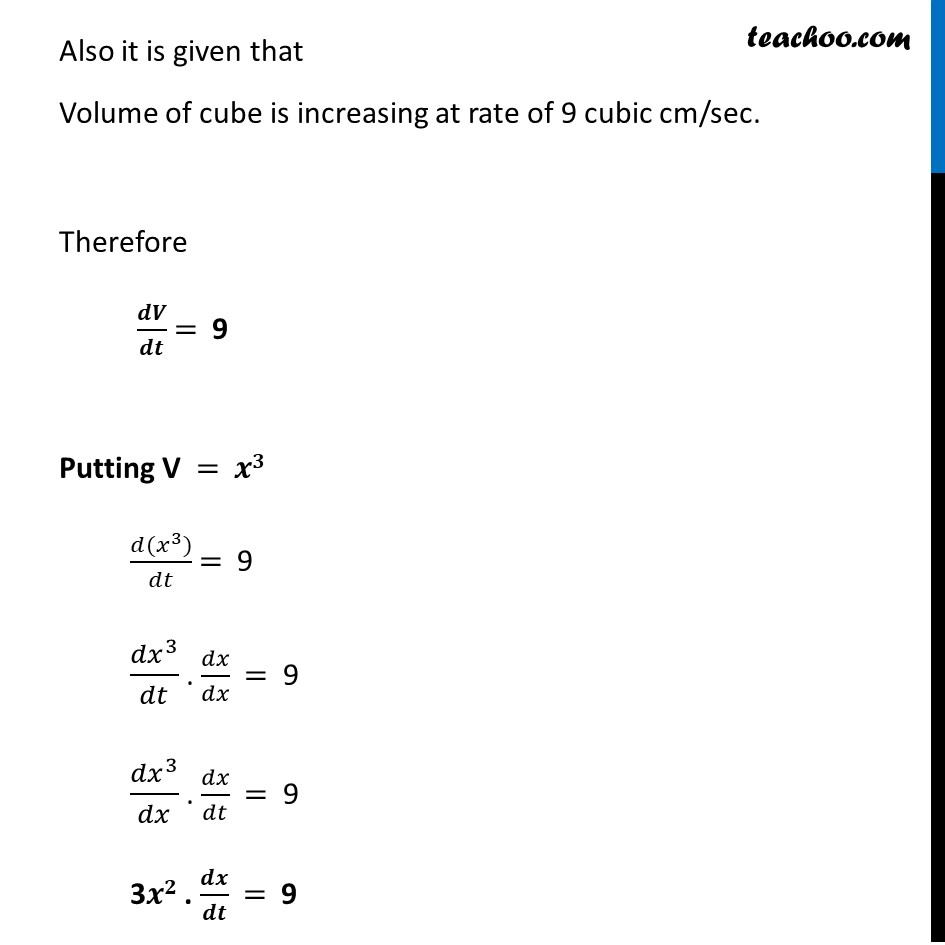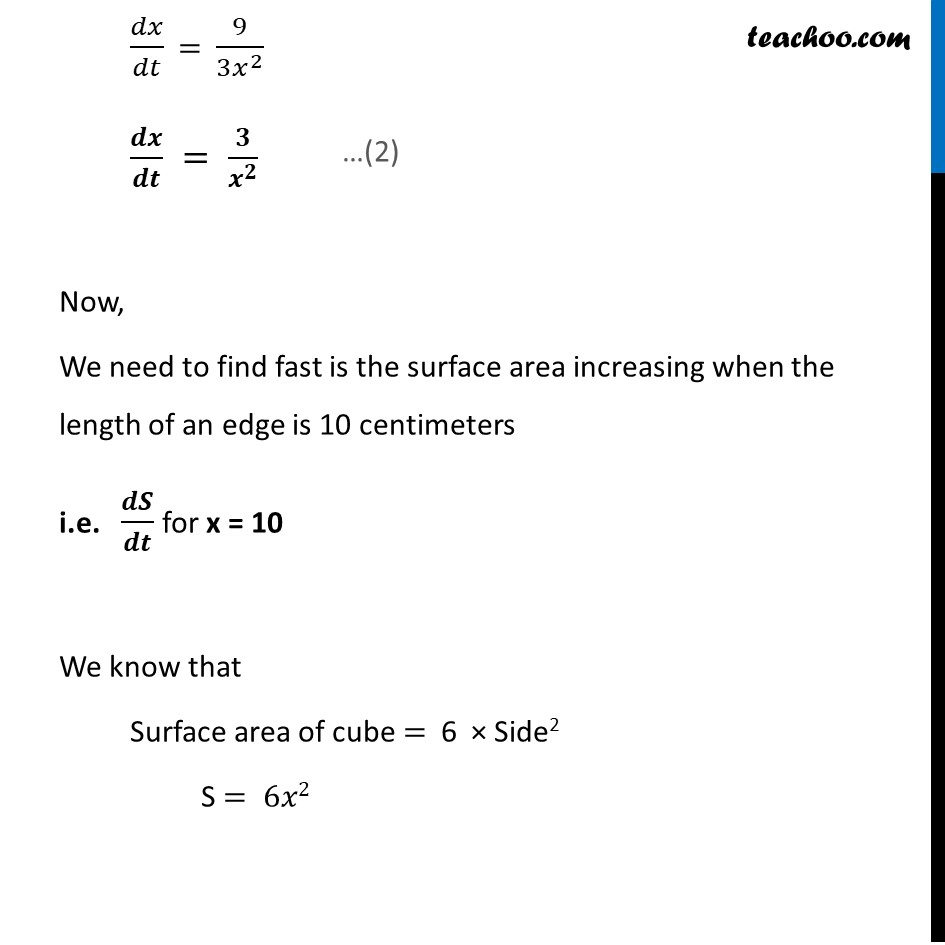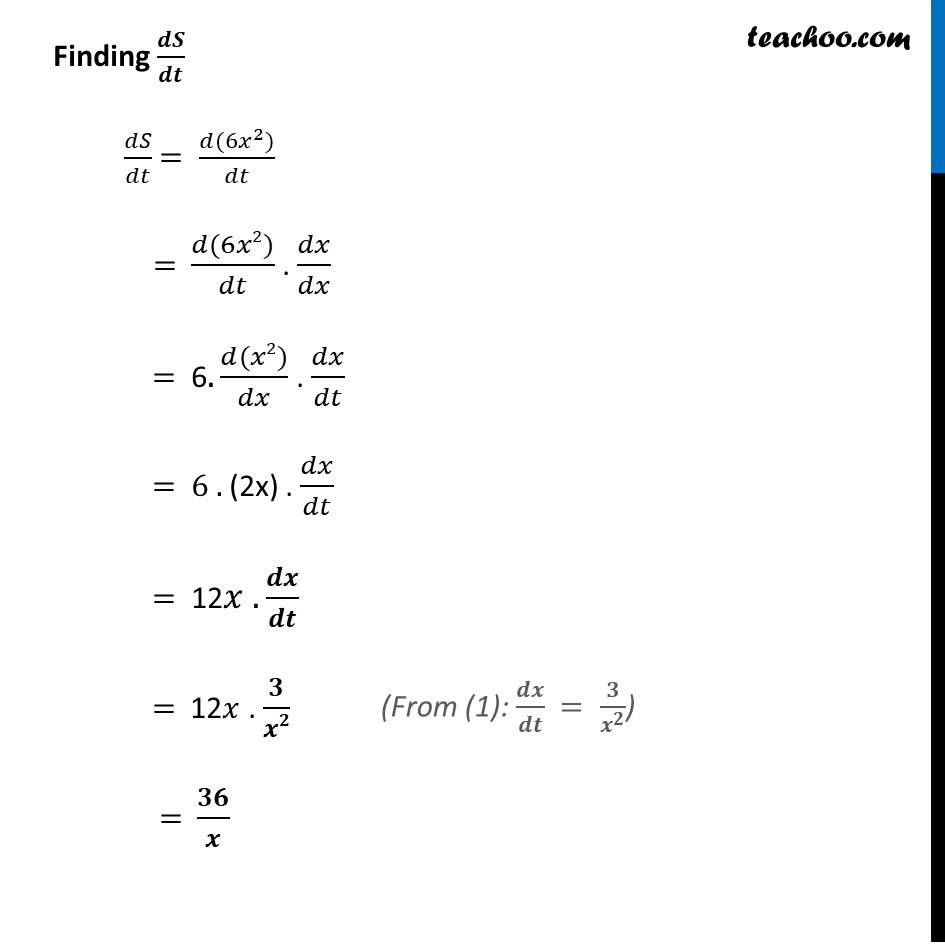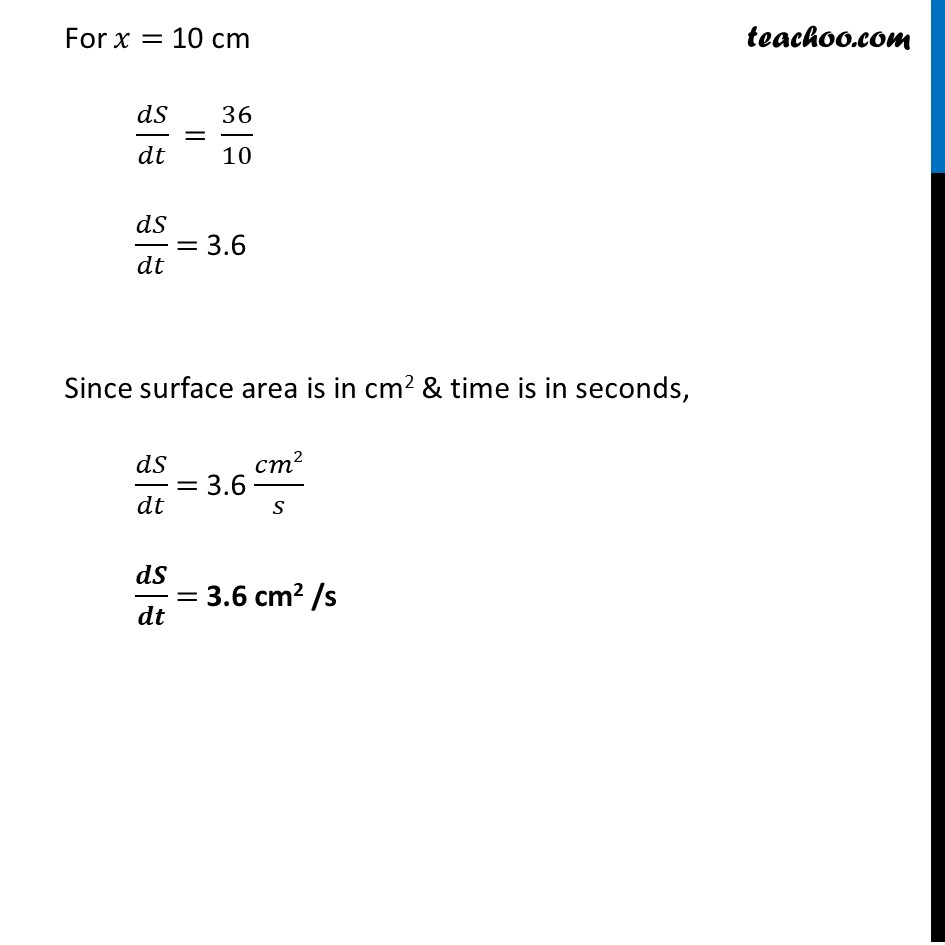Learn in your speed, with individual attention - Teachoo Maths 1-on-1 Class

### Transcript

Example 2 The volume of a cube is increasing at a rate of 9 cubic centimeters per second. How fast is the surface area increasing when the length of an edge is 10 centimeters ?Let 𝒙 be length of side V be Volume t be time per second We know that Volume of cube = (side)3 V = 𝒙𝟑 Also it is given that Volume of cube is increasing at rate of 9 cubic cm/sec. Therefore 𝒅𝑽/𝒅𝒕 = 9 Putting V = 𝒙𝟑 (〖𝑑(𝑥〗^3))/𝑑𝑡 = 9 〖𝑑𝑥〗^3/𝑑𝑡 . 𝑑𝑥/𝑑𝑥 = 9 〖𝑑𝑥〗^3/𝑑𝑥 . 𝑑𝑥/𝑑𝑡 = 9 3𝒙𝟐 . 𝒅𝒙/𝒅𝒕 = 9 𝑑𝑥/𝑑𝑡 = 9/〖3𝑥〗^2 𝒅𝒙/𝒅𝒕 = 𝟑/𝒙^𝟐 Now, We need to find fast is the surface area increasing when the length of an edge is 10 centimeters i.e. 𝒅𝑺/𝒅𝒕 for x = 10 We know that Surface area of cube = 6 × Side2 S = 6𝑥2 Finding 𝒅𝑺/𝒅𝒕 𝑑𝑆/𝑑𝑡 = (𝑑(6𝑥^2))/𝑑𝑡 = (𝑑(6𝑥2))/𝑑𝑡 . 𝑑𝑥/𝑑𝑥 = 6. (𝑑(𝑥2))/𝑑𝑥 . 𝑑𝑥/𝑑𝑡 = 6 . (2x) . 𝑑𝑥/𝑑𝑡 = 12𝑥 . 𝒅𝒙/𝒅𝒕 = 12𝑥 . 𝟑/𝒙𝟐 = 𝟑𝟔/𝒙 (From (1): 𝒅𝒙/𝒅𝒕 = 𝟑/𝒙^𝟐 ) For 𝑥= 10 cm 𝑑𝑆/𝑑𝑡 = 36/10 𝑑𝑆/𝑑𝑡 = 3.6 Since surface area is in cm2 & time is in seconds, 𝑑𝑆/𝑑𝑡 = 3.6 𝑐𝑚2/𝑠 𝒅𝑺/𝒅𝒕 = 3.6 cm2 /s